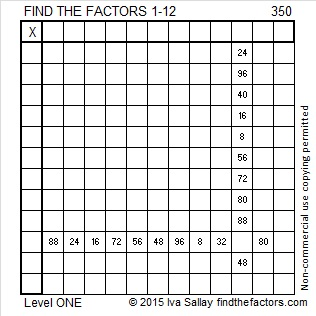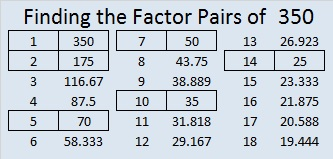# 350 and Level 1

350 is even and thus divisible by 2, so 350 is a composite number. Scroll down past the puzzle to see its factors.

In pose-a-puzzle Ed Southall (solvemymaths) showed examples and described several great mathematical puzzles that are available on the internet. He wrote, “Not only do they excite students in a way that perhaps no textbook page can, they also make you think. I don’t think any good puzzles can be done just by applying a mindless algorithm to a familiar looking structure.”

It will take a while, but check out all the different types of puzzles listed. Here’s something he wrote specifically about the Find the Factors puzzles: “They are the best way I’ve found to get students of *any* ability to practice multiplication tables.”Print the puzzles or type the factors on this excel file: 12 Factors 2015-01-12

• 350 is a composite number.
• Prime factorization: 350 = 2 x 5 x 5 x 7, which can be written 350 = 2·5²·7
• The exponents in the prime factorization are 1, 2, and 1. Adding one to each and multiplying we get (1 + 1)(2 + 1)(1 + 1) = 2 x 3 x 2 = 12. Therefore 350 has exactly 12 factors.
• Factors of 350: 1, 2, 5, 7, 10, 14, 25, 35, 50, 70, 175, 350
• Factor pairs: 350 = 1 x 350, 2 x 175, 5 x 70, 7 x 50, 10 x 35, or 14 x 25
• Taking the factor pair with the largest square number factor, we get √350 = (√14)(√25) = 5√14 ≈ 18.708This site uses Akismet to reduce spam. Learn how your comment data is processed.